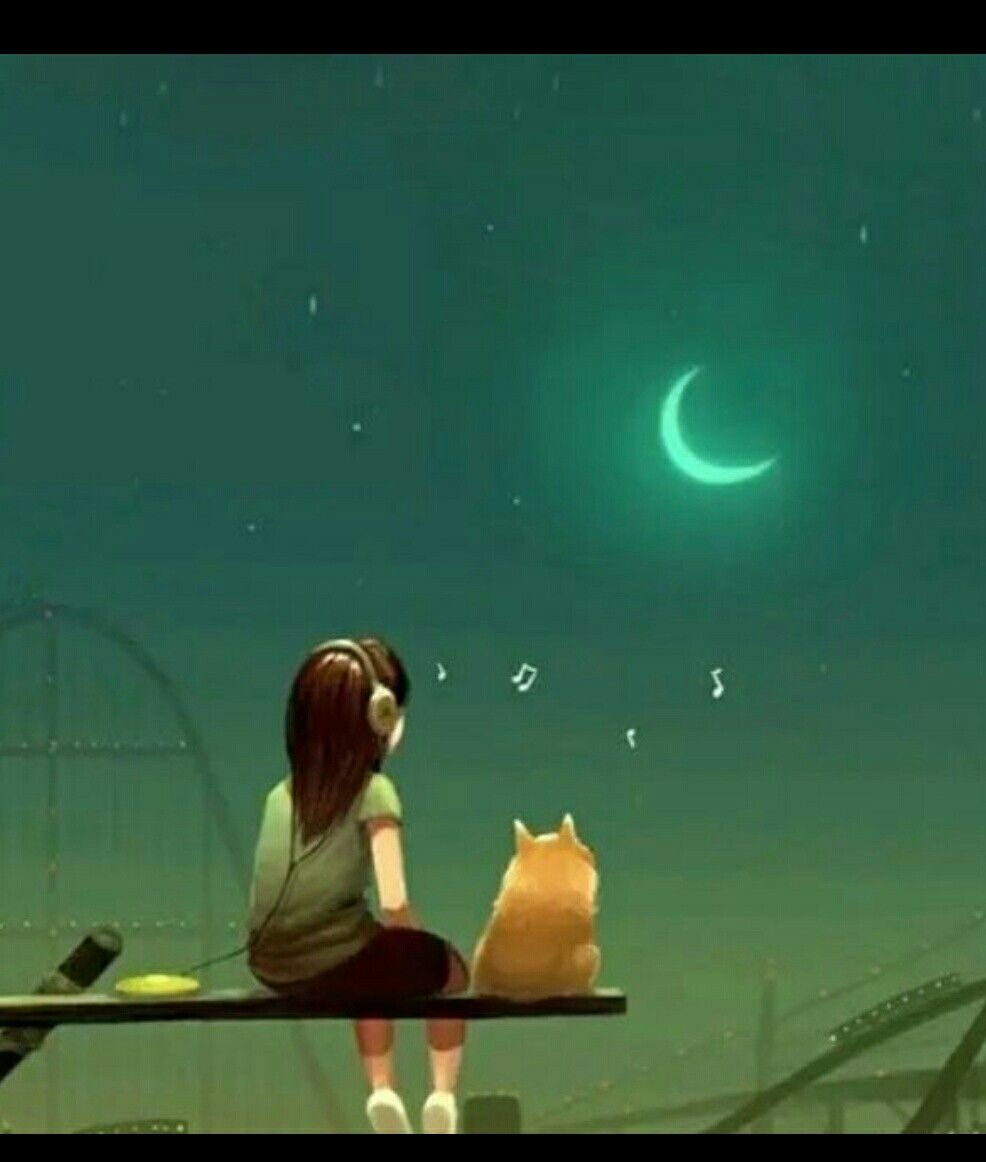Symbol
Problem-----1. The greatest common factor of 6x?y3-12x³y2 +18xty is A. 2xy2 B. 6xy C. 3xy D. 6x-y? 2. What is the greatest common monomial factor of 9xy-54xy? D. 6xy A. 9 B. xy CC. . 98xx y 3. What is the greatest common monomial factor of the expression $16x^{2}-8xy-24xy^{3}7$ A. 4 B. 8 D.4xy 4. Express in factored form, the binomial 2xy-4xy³ is equivalent to: A. (x-2y) B. 2xy (x-2y?) D. 2xy (y-2) A2. ox5y f xt2h-e 9 factors of 25x2 -9 is: 5x-3. Find the other factor. 5. One B. 5x - 3 CCC. . . 254x 9xy -+(93 xyp -q 4y) D. 5x? +9 6. Which of the following is not factorable? A. 4a? - 49 B. 36x2- 25y D. none of the above 7. The expression m8 - nº is equal to (m?)3 - (n) 3 D. (n-2)3 A. (n2)3 B. (n)3 C. 8. What is the value of K in x2-kx+ 144 to make it a perfect square trinomial? A. 12 B. 24 C. 48 D. 72 9. Which of the following values of K will make y? -8y + K factorable? A. 4. B. 12 C. 16 D. 24 10. Which of the following is a perfect square trinomial? y?-10y+25 A. Y2-4 B. y? + 81 y?-16y-64 D. 11. If the area of the square is y? -22y + 121, What is the length of the CsCid. . e of the square? A. Y+11 B. y - 11 y+8 D. Y +3 12. If the area of a square garden is (9x2 -6x + 1) square units, is it possible to solve the sides? A. Yes, using factoring difference of two squares. C. No, one of the sides must be given. B. Yes, the area is a perfect square trinomial. D. No, the area is not factorable. 13. What is the factor of polynomial x2 + 6x +8? (x+9 D. (x- 1) A. (x+3) (x+ 2) B. (x + 2) (x+4) $C\left(x+8\right)\left(x-2\right)$ $=2x^{2+15x+1}c^{8}$ $.118$ 14. Find the missing tem: (x + )(2x + A. 6, 3 B. 9, 2 D. 3, 5 +) 15. Find the missing values in the factors of the polynomial 3y2 + 14y+8 = (3y+(y A. 1,8 B. 8, 1 C. 2, 4 16. If one of the factors of y2 - 6y – 55 is y + 5, what is the other factor? A. Y-5 B. y - 11 C. y+ 11 $0.4.2$ $0.y.1$ $0.\left(y+6\right)\left(y+3\right)$ 17. What is the factor of the expression y2 -9y + 18? A. (Y-6) (y-3) B. (y-6) (rya+is3e) d to 0? $c.\left(y+6\right)\left(y-3\right)$ 18. What is the value of a non-zero polynomial C. A. Zero B. One 19. What expression could represent the length the rectangle Uw(h10ony dse- fe in1e) d (y + is 21) 0y² – 19y-2? determined area DD. . (C10ay nn+ ot 1) (y+2) A. (10y-1) (y- 2) B. (10y + + 1) a_(nyd ) - (w22ix d) t+ h of C. 20. Find the missing term: 2x2 + 15x + 18 = (x A. 6,3 B. 9, 2 C. 1, 18 D. 3, 5 21. The factors of the expressions x2-4x (x+6) (x-4) B. (x-+624x) -(x2- 4 a1r) e C. (x - 3) (x + 8) $0.\left(x-12\right)\left(x+2\right)$ 2A2. . Factor xy + x + 2y + 2 A. ((9y+ a-c81o) 71ma() X2p(-9l+ a4et-29 e) l7y: ) B. (x + 1) (y + 2) $C.\left(x+2\right)\left(y+2\right)$ $D.\left(y+1\right)\left(x+1\right)$ 23. Factor A. B. (9a + 7) (9a – 7) $C.\left(9a+7\right)\left(9a+7\right)$ $D.\left(7a-9\right)\left(7a+9\right)$ 24. Factor completely: x3 + x2 – x-1. $c.\left(x+1\right)\left(x+1\right)\left(x-1\right)$ $D.\left(x-1\right)\left(x-1\right)\left(x+1\right)$ A. (x2 + 1) 9x- 1) 25. What is the factored form of the expression BoBB. f . . x(((x 2 X3y-+ -- 513x ) 6) z(+ x() 92x y- 6(2+ 3? -y21-3) ) 66z2z) ? $c.\left(3y+6z\right)\left(3y+6z\right)$ $D.\left(6y-3z\right)\left(6y+3z\right)$ A. (3y +6z) (3y-6z) 26. Which of the following is a factor C. (x- 3) (x– 2) A. (X+3) (X+ 2) A. (3x- 4y)2 $B.\left(3x-4y\right)\left(3x+4y\right)$ ) C. (3x +4y)2 $0.\left(x+3\right)\left(x-2\right)$ $0.\left(3x+4\right)\left(3x-4y\right)$ $0\left(x-9\right)\left(x-9\right)$ 27. Factor 9x2 - 24xy + 16y2 28. Factor x2+ 18x + 81 A. (x - 9)2 B. (x + 9)2 CF. ind (x+t9h) e (xsi-d9e ) of the parking lot. 29. A square parking area has an area equal to 100x2 -80x + 16 meters. A. (10x + 4)2 B. (50x-8)2 C. (10x- 4)2 D. (50x + 8)2 30. A Square painting is surrounded by a 3-centimeter-wide frame. If the total area of the painting plus frame is 961 cm2, find the dimensions of the painting in factored form. $B.\left(x+25\right)\left(x-37\right)$ $c.\left(x-25\right)\left(x-37\right)$ $D.\left(x+25\right)\left(x+37\right)$ A. (x-25) (x + 37) Prepared by:
7th-9th grade
Algebra
Search count: 124
SolutionQanda teacher - saikumar
tooooo many questions in one pic send one only